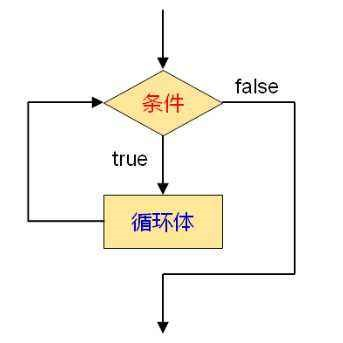（1）用goto语句和if语句构成循环；

（2）用while语句；

（3）用do-while语句；

（4）用for语句；

1、goto语句及goto语句构成循环

goto语句是一种无条件转移语句。使用格式为：goto 语句标号；

```#include<stdio.h>

int main()
{
int i,sum=0;
i=1;
loop:if(i<=100)
{
sum=sum+i;
i++;
goto loop;
}
printf("%d\n",sum);
}```

while语句

while语句一般形式为：while（表达式）语句

while语句语义为：计算表达式的值，当值为真（非0）时，执行循环体语句。其执行过程如图：```include<stdio.h>

int main()
{
int i,sum=0;
i=1;
while(i<=100)
{
sum=sum+i;
i++;
}
printf("%d\n",sum);
}```

```#include<stdio.h>

int main()
{
int i,sum=0;
i=1;
while(i<=100)
//{
sum=sum+i;
i++;
//}
printf("%d\n",sum);
}```

```#include<stdio.h>

void main()
{
int n=0;
printf("input a string:\n");
while(getchar()!='\n')
{
n++;
}
printf("%d",n);
}```